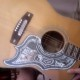### immenent death of the net predicted

1. There are 4 quarts to a gallon, 2 pints to a quart, and 16 ounces to a pint.  There are 4 ounces to a gill.  There are (about) 26.4 gallons in a hectoliter.  How many gills are in a hectoliter?  Display the unit fractions for your calculation.
2.   Write as a single number in scientific notation:  ( 1.5 * 10^{-17} ) / ( 7.5 * 10^8 ).

3.  Consider sets X, Y, and Z in a universal set U, where

U = {b, i, g, f, a, t, c, o, d, e}

X = {e, g, b, d, f}

Y = {f, a, c, e}

Z = {b, e, a, t}.

Use the operations \prime, \cap, \cup—complement, intersection, and union—to find the sets represented by the given expressions.

X \cap Y = { f, e}

Y \cup Z =

Z’ =

(Y \cup Z)’ =

(X \cap Y) \cup Z’ =

X \cap (Y \cup Z)’ =

4. Sum the sequence: 88 + 99 +110 +  . . . + 462.

5.  Expand the expression (x-3)^4.

6.  250 beverages are tested for Purity, Body, and Flavor.

65 pass the Purity test.

95 pass the Body test.

90 pass the Flavor test.

20 pass on both Purity and Body.

25 pass on both Purity and Flavor.

40 pass on both Body and Flavor.

5 pass all three of the tests.

How many of the beverages pass *none* of the tests?

#### 1 Comment

1.vlorbik

the editor on this very mac (not the net) interprets my beginning with “1” as permission to fuck with the fucking formatting. what the fuck, right? it’s like “clippie” hitting you in the face with “would you like to learn about *numbered lists* right now?”—getting out of here, finchley. fuck this forever.

• ## (Partial) Contents Page

Vlorbik On Math Ed ('07—'09)
(a good place to start!)